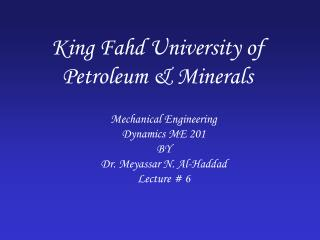Download PresentationKing Fahd University of Petroleum & Minerals

# King Fahd University of Petroleum & Minerals

Download Presentation## King Fahd University of Petroleum & Minerals

- - - - - - - - - - - - - - - - - - - - - - - - - - - E N D - - - - - - - - - - - - - - - - - - - - - - - - - - -
##### Presentation Transcript

1. King Fahd University of Petroleum & Minerals Mechanical Engineering Dynamics ME 201 BY Dr. Meyassar N. Al-Haddad Lecture # 6

2. Objective • To investigate particle motion along a curved path “Curvilinear Motion” using three coordinate systems • Rectangular Components • Position vector r = x i + y j + z k • Velocity v = vx i + vy j + vz k (tangent to path) • Acceleration a = ax i + ay j +az k (tangent to hodograph) • Normal and Tangential Components • Position (particle itself) • Velocity v = u ut (tangent to path) • Acceleration (normal & tangent) • Polar & Cylindrical Components

3. Curvilinear Motion: Cylindrical Components • Section 12.8 • Observed and/or guided from origin or from the center • Cylindrical component • Polar component “plane motion”

4. Application: Circular motion but observed and/or controlled from the center

5. Polar Coordinates • Radial coordinate r • Transverse coordinate • q and r are perpendicular • Theta q in radians • 1 rad = 180o/p • Direction ur and uq

6. Position • Position vector • r = r ur

7. Velocity • Instantaneous velocity = time derivative of r • Where

8. Velocity (con.) • Magnitude of velocity • Angular velocity • Tangent to the path • Angle = q + d d

9. Acceleration • Instantaneous acceleration = time derivative of v

10. Acceleration (con.) • Angular acceleration • Magnitude • Direction “Not tangent” • Angle q + f f

11. Cylindrical Coordinates • For spiral motion cylindrical coordinates is used r, q, and z. • Position • Velocity • Acceleration

12. If r = r(t) and q = q(t) If r = f(q) use chain rule Time Derivative to evaluate

13. Problem • The slotted fork is rotating about O at a constant rate of 3 rad/s. Determine the radial and transverse components of velocity and acceleration of the pin A at the instant q = 360o. The path is defined by the spiral groove r = (5+q/p) in., where q is in radians.

14. Example 12-20

15. Problem A collar slides along the smooth vertical spiral rod, r = (2q) m, where q is in radians. If its angular rate of rotation is constant and equal 4 rad/s, at the instant q = 90o. Determine - The collar radial and transverse component of velocity - The collar radial and transverse component of acceleration. - The magnitude of velocity and acceleration

16. Thank You